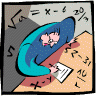## Mathematics, Department of## Dissertations, Theses, and Student Research Papers in Mathematics

#### Authors

Vinodchandran Variyam

5-5-2023

#### Citation

J. Vander Woude, "Partitions of R^n with Maximal Seclusion and their Applications to Reproducible Computation," Ph.D. dissertation, School of Computing & Department of Mathematics, University of Nebraska-Lincoln, Lincoln, NE, USA, 2023.

A DISSERTATION Presented to the Faculty of The Graduate College at the University of Nebraska In Partial Fulfillment of Requirements For the Degree of Doctor of Philosophy, Majors: Mathematics & Computer Science, Under the Supervision of Professors N. V. Vinodchandran & Jamie Radcliffe. Lincoln, Nebraska: May, 2023

This dissertation includes essentially all content from my first author pre-prints Geometry of Rounding and Geometry of Rounding: Near Optimal Bounds and a New Neighborhood Sperner's Lemma and also includes my contributions in the pre-print List and Certificate Complexities in Replicable Learning. This dissertation also includes results not available elsewhere at the time of submission.

#### Abstract

We introduce and investigate a natural problem regarding unit cube tilings/partitions of Euclidean space and also consider broad generalizations of this problem. The problem fits well within a historical context of similar problems and also has applications to the study of reproducibility in randomized computation.

Given $k\in\mathbb{N}$ and $\epsilon\in(0,\infty)$, we define a $(k,\epsilon)$-secluded unit cube partition of $\mathbb{R}^{d}$ to be a unit cube partition of $\mathbb{R}^{d}$ such that for every point $\vec{p}\in\R^d$, the closed $\ell_{\infty}$ $\epsilon$-ball around $\vec{p}$ intersects at most $k$ cubes. The problem is to construct such partitions for each dimension $d$ with the primary goal of minimizing $k$ and the secondary goal of maximizing $\epsilon$.

We prove that for every dimension $d\in\mathbb{N}$, there is an explicit and efficiently computable $(k,\epsilon)$-secluded axis-aligned unit cube partition of $\mathbb{R}^d$ with $k=d+1$ and $\epsilon=\frac{1}{2d}$. We complement this construction by proving that for axis-aligned unit cube partitions, the value of $k=d+1$ is the minimum possible, and when $k$ is minimized at $k=d+1$, the value $\epsilon=\frac{1}{2d}$ is the maximum possible. This demonstrates that our constructions are the best possible.

We also consider the much broader class of partitions in which every member has at most unit volume and show that $k=d+1$ is still the minimum possible. We also show that for any reasonable $k$ (i.e. $k\leq 2^{d}$), it must be that $\epsilon\leq\frac{\log_{4}(k)}{d}$. This demonstrates that when $k$ is minimized at $k=d+1$, our unit cube constructions are optimal to within a logarithmic factor even for this broad class of partitions. In fact, they are even optimal in $\epsilon$ up to a logarithmic factor when $k$ is allowed to be polynomial in $d$.

We extend the techniques used above to introduce and prove a variant of the KKM lemma, the Lebesgue covering theorem, and Sperner's lemma on the cube which says that for every $\epsilon\in(0,\frac12]$, and every proper coloring of $[0,1]^{d}$, there is a translate of the $\ell_{\infty}$ $\epsilon$-ball which contains points of least $(1+\frac23\epsilon)^{d}$ different colors.